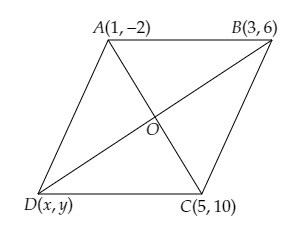# If three consecutive vertices of a parallelogram are $(1, -2), (3, 6)$ and $(5, 10)$, find its fourth vertex.

Given:

Three consecutive vertices of a parallelogram are $(1, -2), (3, 6)$ and $(5, 10)$.

To do:

We have to find the fourth vertex.

Solution:

Let the coordinates of the three vertices are $A (1, -2), B (3, 6)$ and $C (5, 10)$.
Let the fourth vertex be $D(x,y)$ and the diagonals $AC$ and $BD$ bisect each other at $O$.This implies,

$\mathrm{O}$ is the mid-point of $\mathrm{AC}$.

The coordinates of $\mathrm{O}$ are $(\frac{1+5}{2}, \frac{-2+10}{2})$

$=(\frac{6}{2}, \frac{8}{2})$

$=(3,4)$

$\mathrm{O}$ is the mid-point of $\mathrm{BD}$.

The coordinates of $\mathrm{O}$ are $(\frac{3+x}{2}, \frac{6+y}{2})$

Therefore,

$(3,4)=(\frac{3+x}{2}, \frac{6+y}{2})$

On comparing, we get,

$\frac{3+x}{2}=3$

$3+x=3(2)$

$x=6-3=3$

Similarly,

$\frac{6+y}{2}=4$

$6+y=4(2)$

$y=8-6=2$

Therefore, the coordinates of the fourth vertex are $(3,2)$.

Updated on: 10-Oct-2022

24 Views